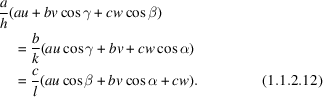International
Tables for
Crystallography
Volume C
Mathematical, physical and chemical tables
Edited by E. Prince

International Tables for Crystallography (2006). Vol. C, ch. 1.1, pp. 3-4

## Section 1.1.2. Lattice vectors, point rows, and net planes

E. Kocha

aInstitut für Mineralogie, Petrologie und Kristallographie, Universität Marburg, Hans-Meerwein-Strasse, D-35032 Marburg, Germany

### 1.1.2. Lattice vectors, point rows, and net planes

| top | pdf |

The length t of a vectoris given by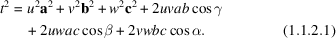Accordingly, the length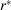of a reciprocal-lattice vector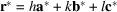may be calculated from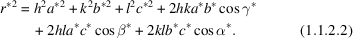If the coefficients u, v, w of a vectorare coprime, [uvw] symbolizes the direction parallel to t. In particular, [uvw] is used to designate a crystal edge, a zone axis, or a point row with that direction.

The integer coefficients h, k, l of a vector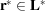are also the coordinates of a point of the corresponding reciprocal lattice and designate the Bragg reflection with scattering vector r*. If h, k, l are coprime, the direction parallel to r* is symbolized by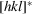.

Each vector r* is perpendicular to a family of equidistant parallel nets within a corresponding direct point lattice. If the coefficients h, k, l of r* are coprime, the symbol (hkl) describes that family of nets. The distance d(hkl) between two neighbouring nets is given by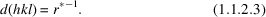Parallel to such a family of nets, there may be a face or a cleavage plane of a crystal.

The net planes (hkl) obey the equation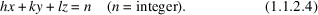Different values of n distinguish between the individual nets of the family; x, y, z are the coordinates of points on the net planes (not necessarily of lattice points). They are expressed in units a, b, and c, respectively.

Similarly, each vectorwith coprime coefficients u, v, w is perpendicular to a family of equidistant parallel nets within a corresponding reciprocal point lattice. This family of nets may be symbolized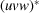. The distance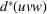between two neighbouring nets can be calculated from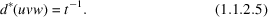A layer line on a rotation pattern or a Weissenberg photograph with rotation axis [uvw] corresponds to one such net of the familyof the reciprocal lattice.

The netsobey the equation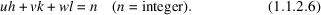Equations (1.1.2.6)and (1.1.2.4)are essentially the same, but may be interpreted differently. Again, n distinguishes between the individual nets out of the family. h, k, l are the coordinates of the reciprocal-lattice points, expressed in units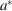,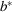,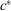, respectively.

A family of nets (hkl) and a point row with direction [uvw] out of the same point lattice are parallel if and only if the following equation is satisfied: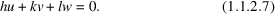This equation is called the `zone equation' because it must also hold if a face (hkl) of a crystal belongs to a zone [uvw].

Two (non-parallel) nets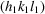and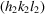intersect in a point row with direction [uvw] if the indices satisfy the condition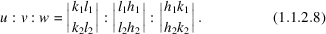The same condition must be satisfied for a zone axis [uvw] defined by the crystal facesand.

Three nets,, and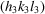intersect in parallel rows, or three faces with these indices belong to one zone if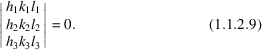Two (non-parallel) point rows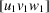and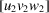in the direct lattice are parallel to a family of nets (hkl) if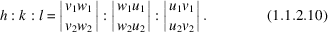The same condition holds for a face (hkl) belonging to two zonesand.

Three point rows,, and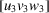are parallel to a net (hkl), or three zones of a crystal with these indices have a common face (hkl) if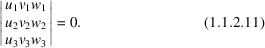A net (hkl) is perpendicular to a point row [uvw] if